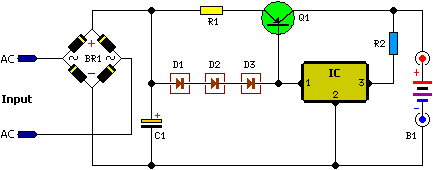12 Volt Car Battery

Parts:

R1 = 0.32R

R2 = 8.2R

C1 = 10,000uF – 63V

D1 = 1N4004

D2 = 1N4004

D3 = 1N4004

Q1 = MJ1504

IC = 7815 REG